# NCERT Solutions for Class 8 Maths Chapter 5 Data Handling Exercise 5.1

NCERT Solutions for Class 8 Maths Chapter 5 Data Handling Exercise 5.1

Ex 5.1 Class 8 Maths Question 1.
For which of these would you use a histogram to show the data?
(i) The number of letters for different areas in a postman’s bag.
(ii) The height of competitors in an athletics meet.
(iii) The number of cassettes produced by 5 companies.
(iv) The number of passengers boarding trains from 7 a.m to 7 p.m at a station.
Give a reason for each.
Solution:
(i) The number of areas cannot be represented in class-intervals. So, we cannot use the histogram to show the data.
(ii) Height of competitors can be divided into intervals. So, we can use histogram here.
For example: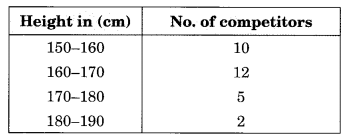(iii) Companies cannot be divided into intervals. So, we cannot use histogram here.
(iv) Time for boarding the train can be divided into intervals. So, we can use histogram here.
For example:Ex 5.1 Class 8 Maths Question 2.
The shoppers who come to a departmental store are marked as: man (M), woman (W), boy (B) or girl (G). The following list gives the shoppers who came during the first hour in the morning.
W W W G B W W M G G M M W W W W
G B M W B G G M W W M M W W W
M W B W G M W W W W G W M M W
W M W G W M G W M M B G G W
Make a frequency distribution table using tally marks. Draw a bar graph to illustrate it.
Solution:Ex 5.1 Class 8 Maths Question 3.
The weekly wages (in ₹) of 30 workers in a factory are:
830, 835, 890, 810, 835, 836, 869, 845, 898, 890,
820, 860, 832, 833, 855, 845, 804, 808, 812, 840,
885, 835, 835, 836, 878, 840, 868, 890, 806, 840
Using tally marks make a frequency table with intervals as 800-810, 810-820 and so on.
Solution: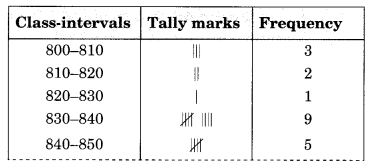Ex 5.1 Class 8 Maths Question 4.
Draw a histogram for the frequency table made for the data in Question 3, and answer the following questions:
(i) Which group has the maximum number of workers?
(ii) How many workers earn ₹ 850 and more?
(iii) How many workers earn less than ₹ 850?
Solution:
Refer to the frequency table of Question No. 3.(i) Group 830-840 has the maximum number of workers, i.e., 9.
(ii) 10 workers earn equal and more than ₹ 850.
(iii) 20 workers earn less than ₹ 850.

Ex 5.1 Class 8 Maths Question 5.
The number of hours for which students of a particular class watched television during holidays is shown through the given graph.
(i) For how many hours did the maximum number of students watch TV?
(ii) How many students watched TV for less than 4 hours?
(iii) How many students spent more than 5 hours watching TV?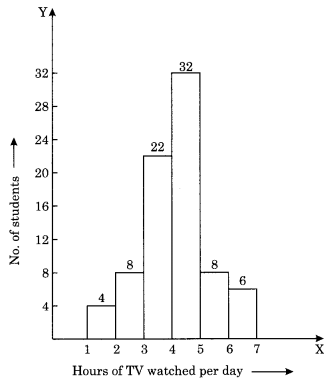Solution:
(i) 32 is the maximum number of students who watched TV for 4 to 5 hours.
(ii) 4 + 8 + 22 = 34 students watched TV for less than 4 hours.
(iii) 8 + 6 = 14 students watched TV for more than 5 hours.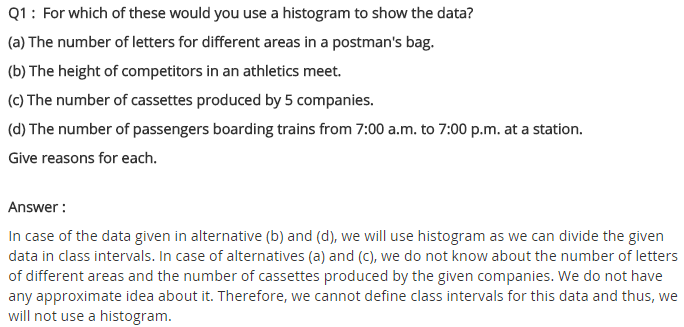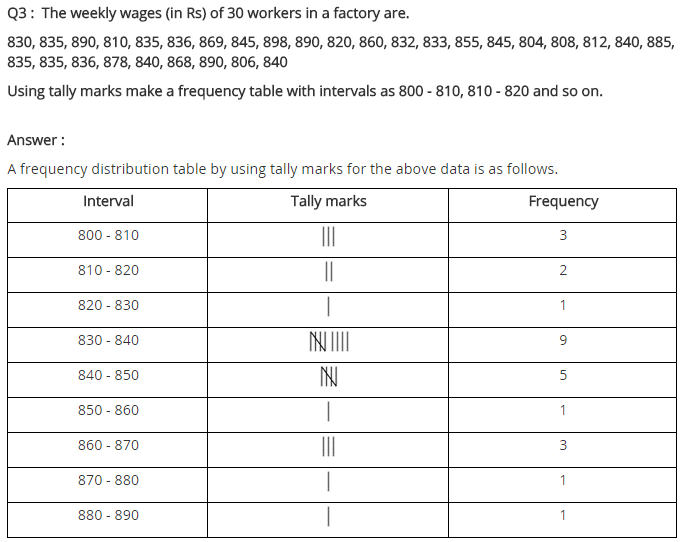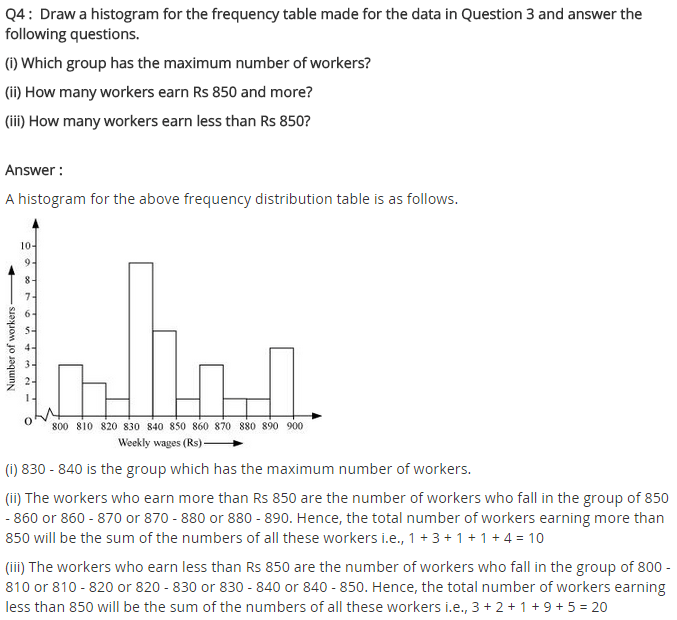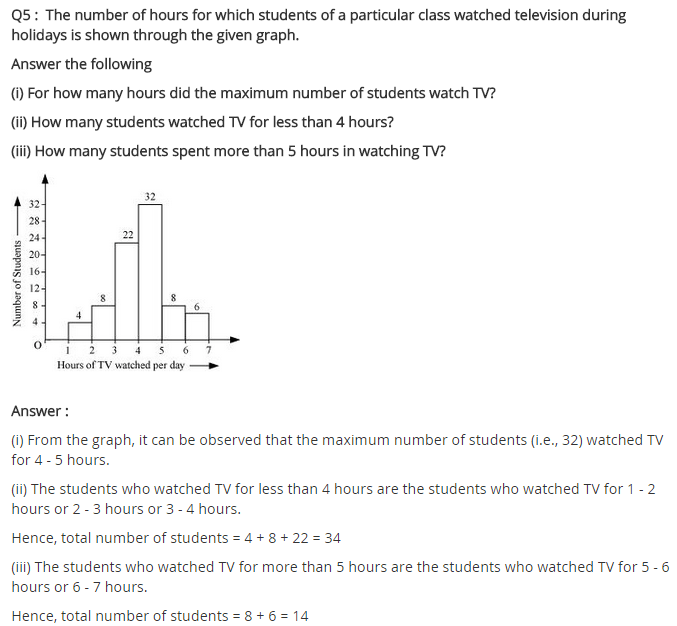+